Courses

# Runoff & Infiltration - 2 Civil Engineering (CE) Notes | EduRev

## Civil Engineering (CE) : Runoff & Infiltration - 2 Civil Engineering (CE) Notes | EduRev

The document Runoff & Infiltration - 2 Civil Engineering (CE) Notes | EduRev is a part of the Civil Engineering (CE) Course Engineering Hydrology.
All you need of Civil Engineering (CE) at this link: Civil Engineering (CE)

Effective rainfall

A part of the rainfall reaching the earth’s surface infiltrates into the ground and finally joins the ground water reservoirs or moves laterally as interflow. Of the interflow, only the quick response or prompt interflow contributes to the immediate rise of the stream flow hydrograph. Hence, the rainfall component causing perceptible change in the stream flow is only a portion of the total rainfall recorded over the catchment. This rainfall is called the effective rainfall.

The infiltration capacity varies from soil to soil and is also different for the same soil in its moist and dry states. If a soil is initially dry, the infiltration rate (or the infiltration capacity of the soil) is high. If the precipitation is lower than the infiltration capacity of the soil, there will be no overland flow, though interflow may still occur. As the rainfall persists, the soil become moist and infiltration rate decreases, causing the balance precipitation to produce surface runoff. Mathematical representation of the infiltration capacity and the methods to deduct infiltration for finding effective rainfall is described later in this lesson.

Methods of base flow separation

Consider the total runoff hydrograph shown in Figure 3, for which the corresponding effective rainfall hyetograph over the catchment is known. In this example, the flow in the stream starts rising at about 4 hours, and the peak is seen to reach at about 10.5 hours. The direct runoff is presumed to end at about 19.5 hours. Though we have separately shown the base flow and the direct runoff in Figures 4 and 5, it is only a guess, as what is observed flowing in the stream is the total discharge. A couple of procedures are explained in the following sub-sections to separate the two flows. For this, we consider another hydrograph (Figure 6), where the total flow is seen to be reducing initially, and then a sudden rise takes place, probably due to a sudden burst of rainfall.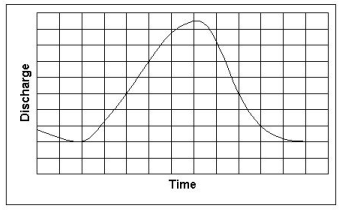FIGURE 6. A typical hydrograph requiring base flow separation

Method 1 One method to separate the base flow from the total runoff hydrograph is to join points X and Z as shown in Figure 7. This method is considered not very accurate, though.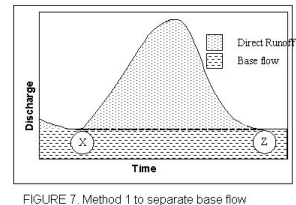Method 2 This method suggests the extension of the base flow graph (Figure 8) along its general trend before the rise of the hydrograph up to a point P directly below the runoff hydrograph peak. From P, a straight line PQ is drawn to meet the hydrograph at point Q, which as separated from P in the time scale by an empirical relation given as:

N (in days) = 0.862 A0.2                        (1)

Where, A is the area of the drainage basin in square kilometers.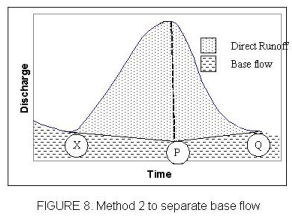Method 3 The third method makes use of composite base flow recession curve, as shown in Figure 9. The following points are to be kept in mind:

X – A follows the trend of the initial base flow recession curve prior to the start of the direct runoff hydrograph

B – Q  follows the trend of the later stage base flow recession curve.

B is chosen to lie below the point of inflection (C) of the hydrograph.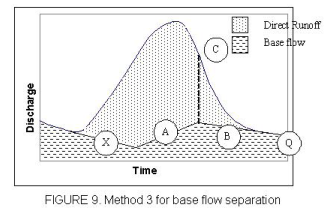The hydrograph after separating and the base flow results in what is called the Direct Runoff Hydrograph.

Estimation of infiltration

The rate at which water infiltrates into a ground is called the infiltration capacity. When a soil is dry, the infiltration rate is usually high compared to when the soil is moist. For an initially dry soil subjected to rain, the infiltration capacity curve shows an exponentially decaying trend as shown in Figure 10. The observed trend is due to the fact that when the soil is initially dry, the rate of infiltration is high but soon decreases, as most of the soil gets moist. The rate of infiltration reaches a uniform rate after some time.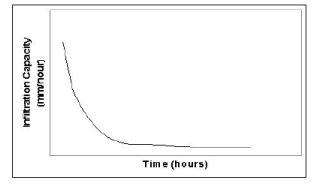FIGURE 10. Infiltration rate decreasing as more water infiltrates

Interestingly, if the supply of continuous water from the surface is cutoff, then the infiltration capacity starts rising from the point of discontinuity as shown in below.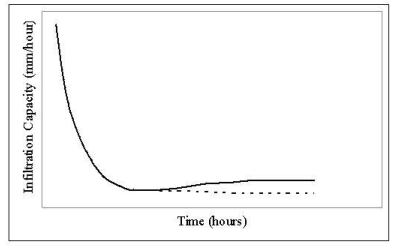FIGURE 11. Infiltration capacity rising after supply from top is cut off

For consistency in hydrological calculations, a constant value of infiltration rate for the entire storm duration is adopted. The average infiltration rate is called the Infiltration Index and the two types of indices commonly used are explained in the next section.

Infiltration indices

The two commonly used infiltration indices are the following:

φ – index

W – index

The φ - index

This is defined as the rate of infiltration above which the rainfall volume equals runoff volume, as shown in Figure 12.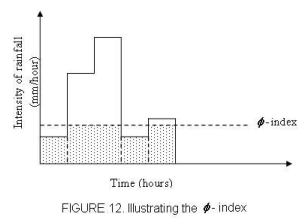The method to determine the - index would usually involve some trial. Since the infiltration capacity decreases with a prolonged storm, the use of an average loss rate in the form of - index is best suited for design storms occurring on wet soils in which case the loss rate reaches a final constant rate prior to or early in the storm. Although the - index is sometimes criticized as being too simple a measure for infiltration, the concept is quite meaningful in the study of storm runoff from large watersheds. The evaluation of the infiltration process is less precise for large watersheds. The data is never sufficient to derive an infiltration curve. Under the circumstances, the - index is the only feasible alternative to predict the infiltration from the storm.

The W – index

This is the average infiltration rate during the time when the rainfall intensity exceeds the infiltration rate.

Thus, W  may be mathematically calculated by dividing the total infiltration (expressed as a depth of water) divided by the time during which the rainfall intensity exceeds the infiltration rate. Total infiltration may be fund out as under:

Total infiltration = Total precipitation – Surface runoff – Effective storm retention

The W – index can be derived from the observed rainfall and runoff data. It differs from the - index in that it excludes surface storage and retention. The index does not have any real physical significance when computed for a multiple complex watershed. Like the phi-index the - index, too is usually used for large watersheds.

Offer running on EduRev: Apply code STAYHOME200 to get INR 200 off on our premium plan EduRev Infinity!

## Engineering Hydrology

17 videos|21 docs|17 tests

,

,

,

,

,

,

,

,

,

,

,

,

,

,

,

,

,

,

,

,

,

;